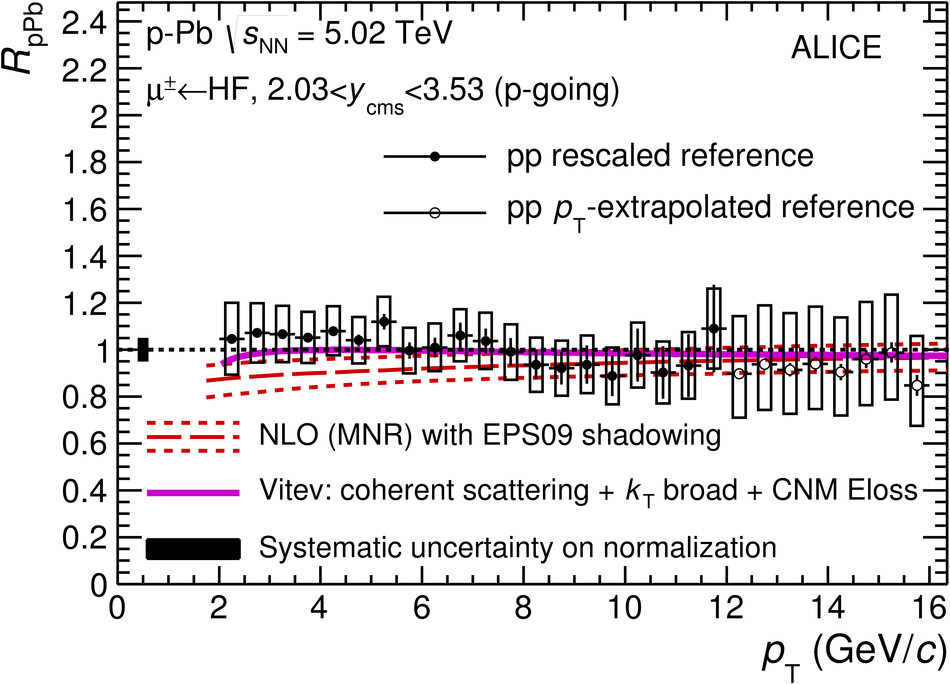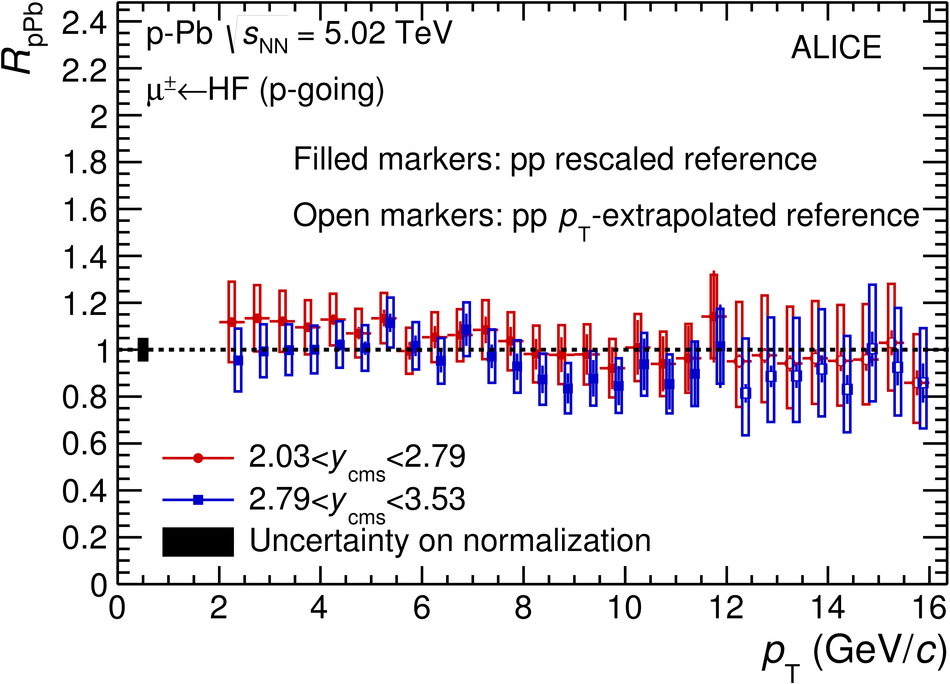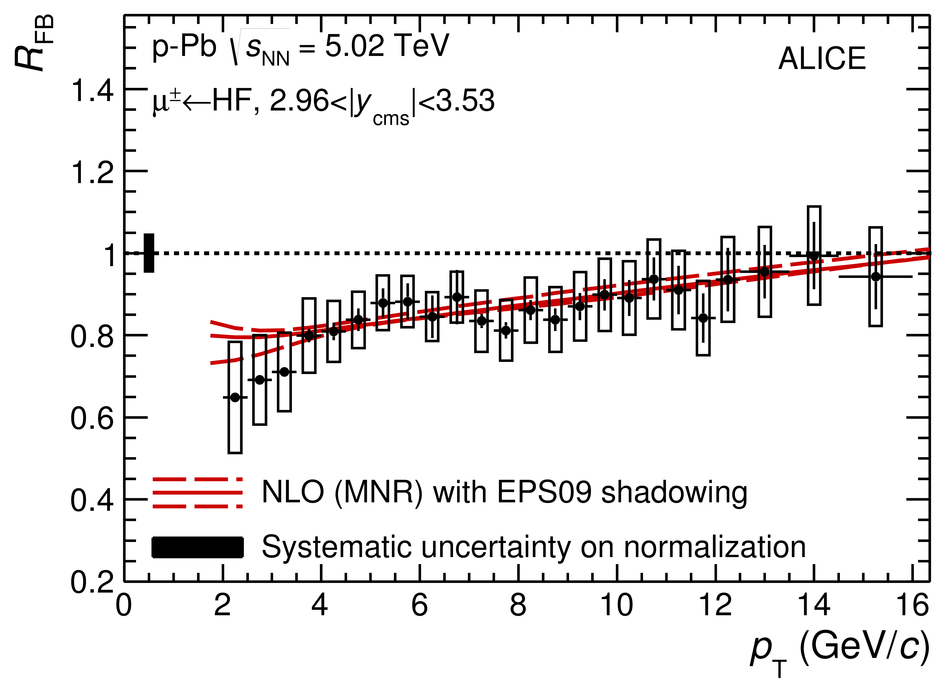Production of muons from heavy-flavour hadron decays in p-Pb collisions at $\sqrt{s_{\rm NN}} = 5.02$ TeV

The production of muons from heavy-flavour hadron decays in p-Pb collisions at $\sqrt{{\textit s}_{\rm NN}}=5.02$ TeV was studied for $2 <~ p_{\rm T} <~ 16$ GeV/$c$ with the ALICE detector at the CERN LHC. The measurement was performed at forward (p-going direction) and backward (Pb-going direction) rapidity, in the ranges of rapidity in the center-of-mass system (cms) $2.03<~y_{\rm cms}<~3.53$ and $-4.46<~y_{\rm cms}<~-2.96$, respectively. The production cross sections and nuclear modification factors are presented as a function of transverse momentum ($p_{\rm T}$). At forward rapidity, the nuclear modification factor is compatible with unity while at backward rapidity, in the interval $2.5<~p_{\rm T}<~3.5$ GeV/$c$, it is above unity by more than 2$\sigma$. The ratio of the forward-to-backward production cross sections is also measured in the overlapping interval $2.96 <~ \vert y_{\rm cms} \vert <~ 3.53$ and is smaller than unity by 3.7$\sigma$ in $2.5<~p_{\rm T}<~3.5$ GeV/$c$. The data are described by model calculations including cold nuclear matter effects.

Figures

Figure 1

 Production cross sections of muons from heavy-flavour hadrondecays as a function of $\pt$ for p-Pb collisions at $\sqrt {s_{\rm NN}}$ = 5.02 TeV at forward rapidity ($2.03 < y_{\rm cms} < 3.53$) and backward rapidity ($-4.46 < y_{\rm cms} < -2.96$). Statistical uncertainties (bars) and systematic uncertainties (boxes) are shown.Figure 2

 Nuclear modification factor of muons from heavy-flavour hadron decays as a function of $\pt$ for p-Pb collisions at $\sqrt {s_{\rm NN}}$ = 5.02 TeV at forward rapidity ($2.03< y_{\rm cms}< 3.53$, top) and backward rapidity ($-4.46< y_{\rm cms}< -2.96$, bottom) compared to model predictions [70-72] Statistical uncertainties (bars), systematic uncertainties (open boxes), and normalisation uncertainties (filled box at $R_{\rm pPb}^{\mu^\pm \leftarrow {\rm HF}}$ = 1) are shown. Filled (open) symbols refer to the pp reference obtained from an energy and rapidity scaling to the measurement at $\sqrt s$ = 7 TeV (an extrapolation based on FONLL calculations).Figure 3

 Nuclear modification factors of muons from heavy-flavour hadron decays as a function of $\pt$ for p-Pb collisions at $\sqrt {s_{\rm NN}}$ = 5.02 TeV in two rapidity sub-intervals at forward (top) and backward (bottom) rapidity. Statistical uncertainties (bars), systematic uncertainties (open boxes), and normalisation uncertainties (filled box at $R_{\rm pPb}^{\mu^\pm \leftarrow {\rm HF}}$ = 1) are shown. For visibility, the points for the rapidity intervals $2.79 < y_{\rm cms} < 3.53$ and $-3.71 < y_{\rm cms} < -2.96$ are slightly shifted horizontally. Filled (open) symbols refer to the pp reference obtained from anenergy and rapidity scaling to the measurement at $\sqrt s$ = 7 TeV (an extrapolation based on FONLL calculations).Figure 4

 Forward-to-backward ratio of muons from heavy-flavour hadron decays as a fucntion of $\pt$ for p-Pb collisions at $\sqrt {s_{\rm NN}}$ = 5.02 TeV compared to model predictions . Statistical uncertainties (bars), systematic uncertainties (open boxes) and normalisation uncertainties (filled box at $R_{\rm FB}^{\mu^\pm \leftarrow {\rm HF}}$ = 1) are shown.